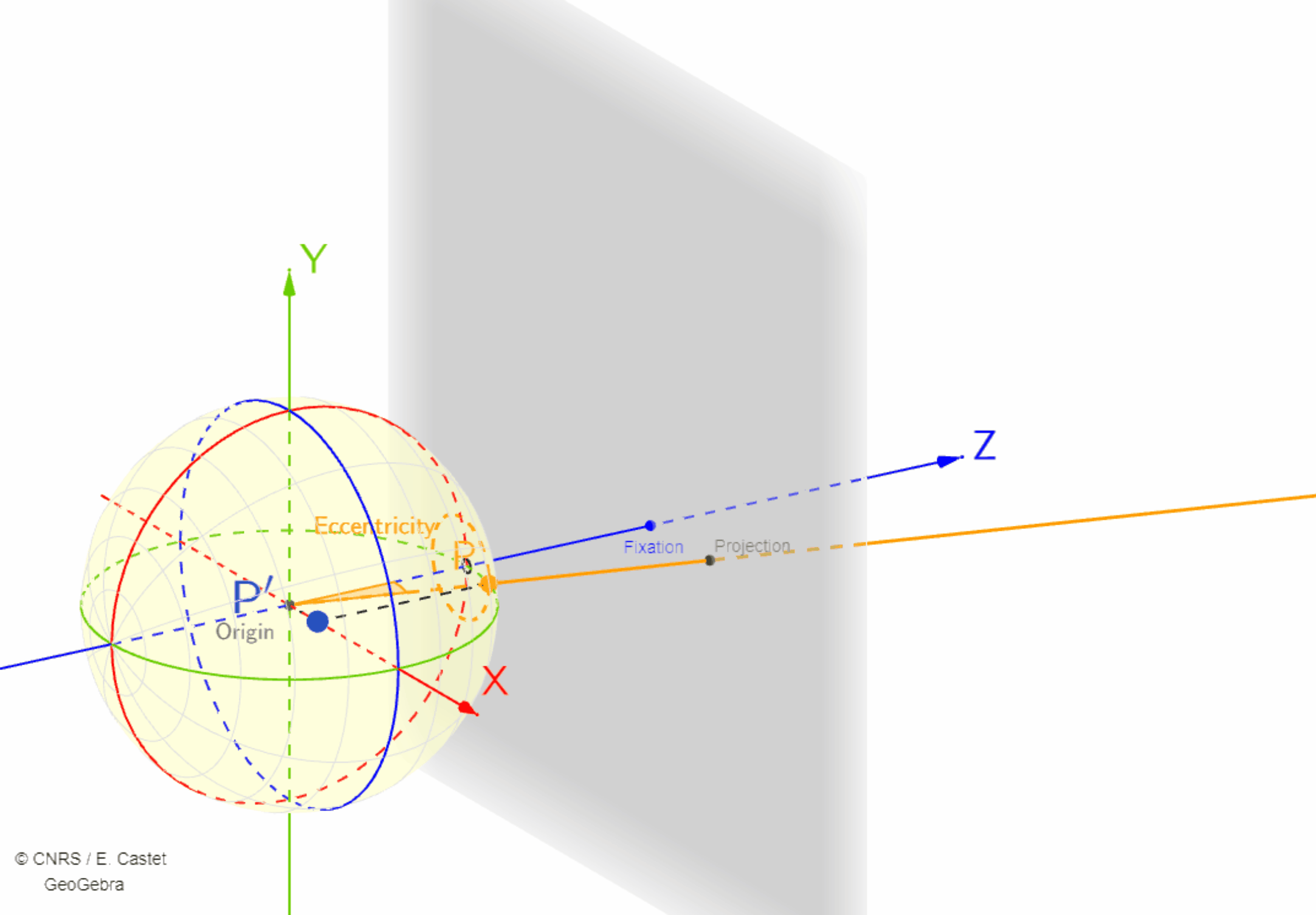# Perimetric coordinate system : Eccentricity and Half-meridian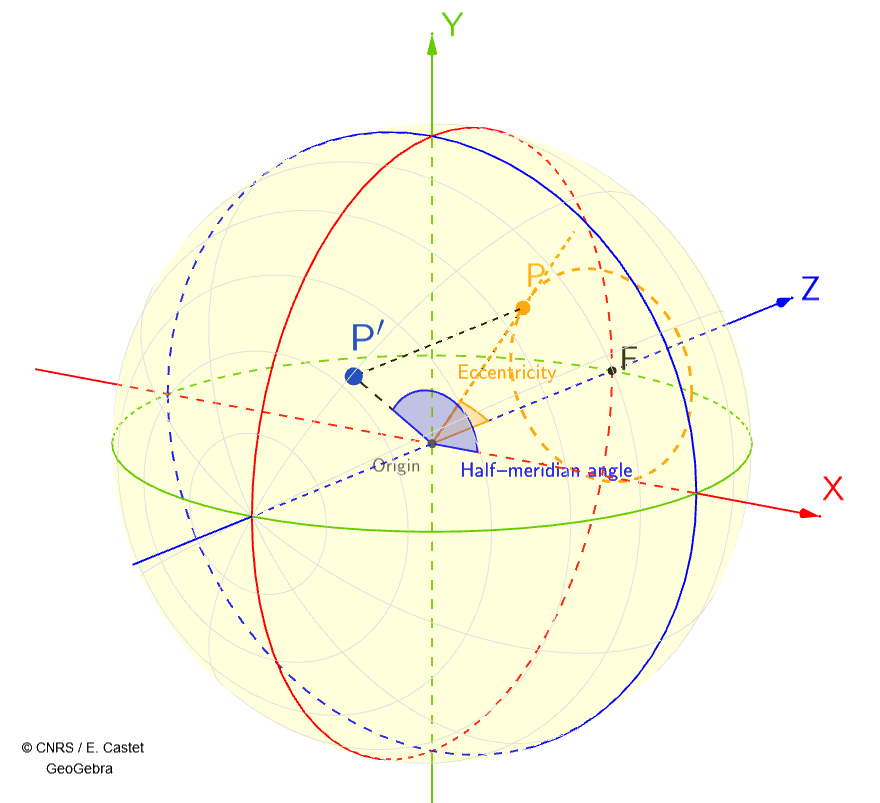Figure 1: Static 3D representation of the perimetric coordinate system.

The PTVR perimetric coordinate system is a spherical coordinate system that is used extensively by vision scientists.

In this coordinate system, the 3D position of a point P is defined by 3 coordinates:
– The Half-meridian (from 0° to 360°).
– The Eccentricity (from 0° to 180°).
– The Radial Distance (in meters) between Origin and P.

The 3 coordinates of point P in Figure 1 are:
Half-meridian: 135°
Eccentricity: 20°
Radial distance: any distance in meters.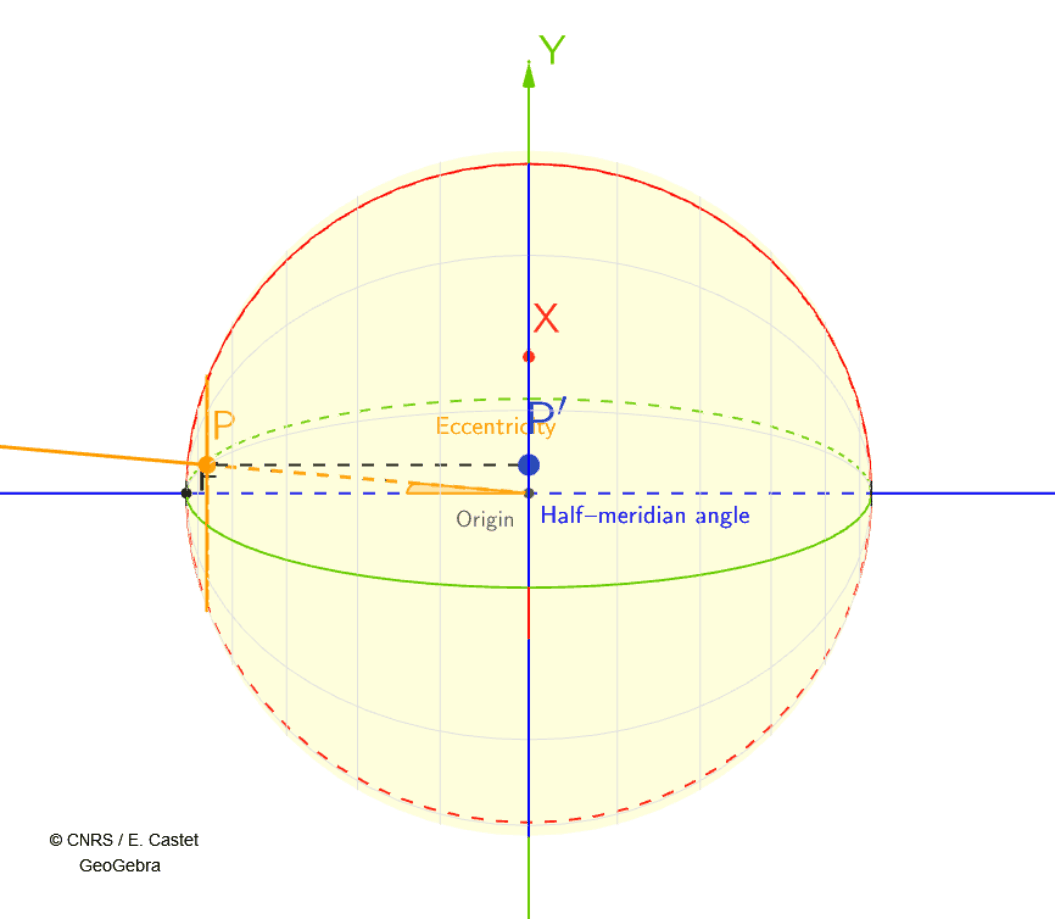Figure 2: Horizontal rotation (i.e. about the Y axis) of the perimetric coordinate system shown in figure 1

A horizontal rotation (i.e. about the Y axis) of the perimetric coordinate system shown in figure 1 is shown in Figure 2. This rotation aims at providing a better mental image of 3D space.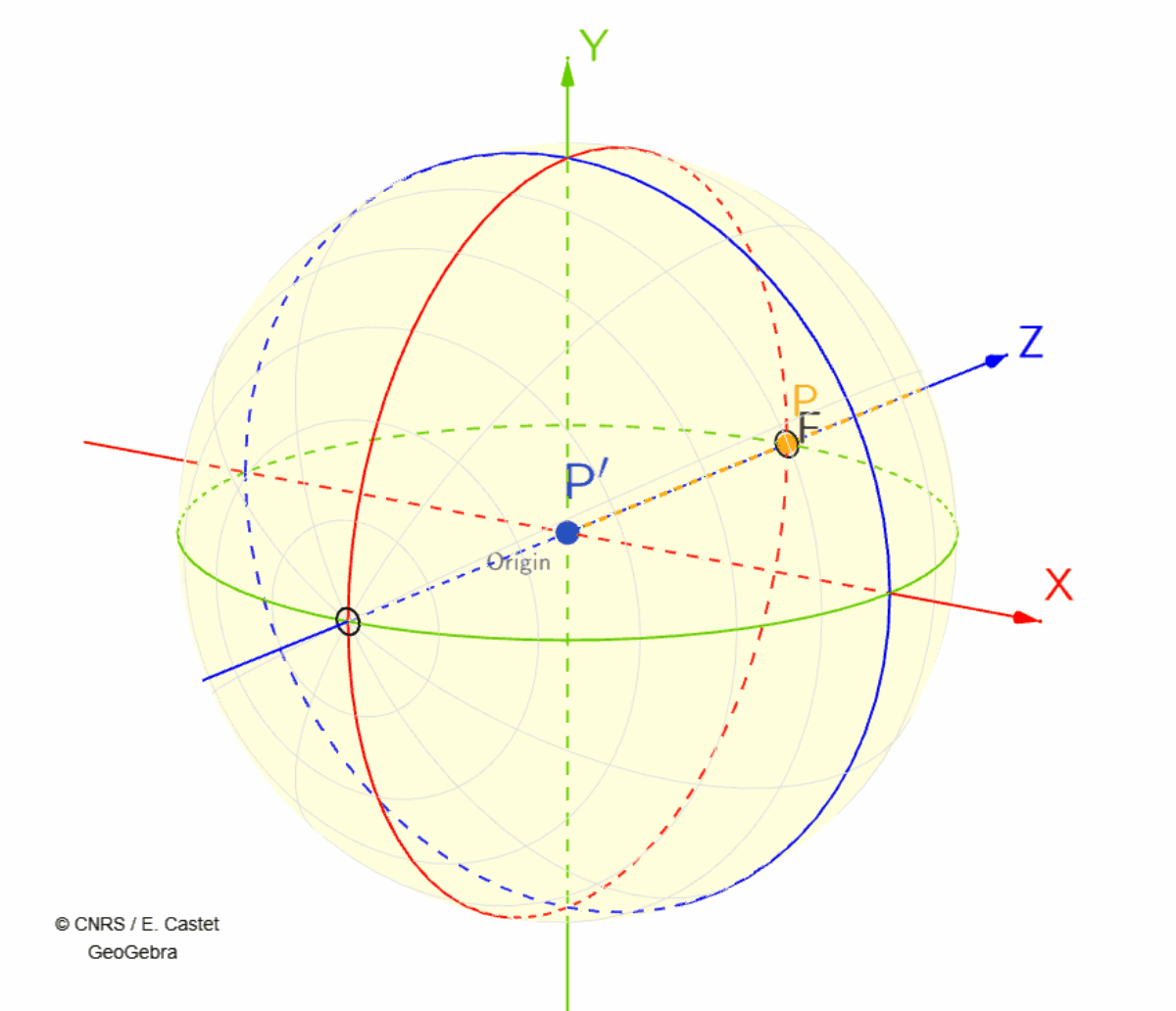Figure 3: Eccentricity of point P varies from 0° to 40° while keeping Half-meridian constant at 135°.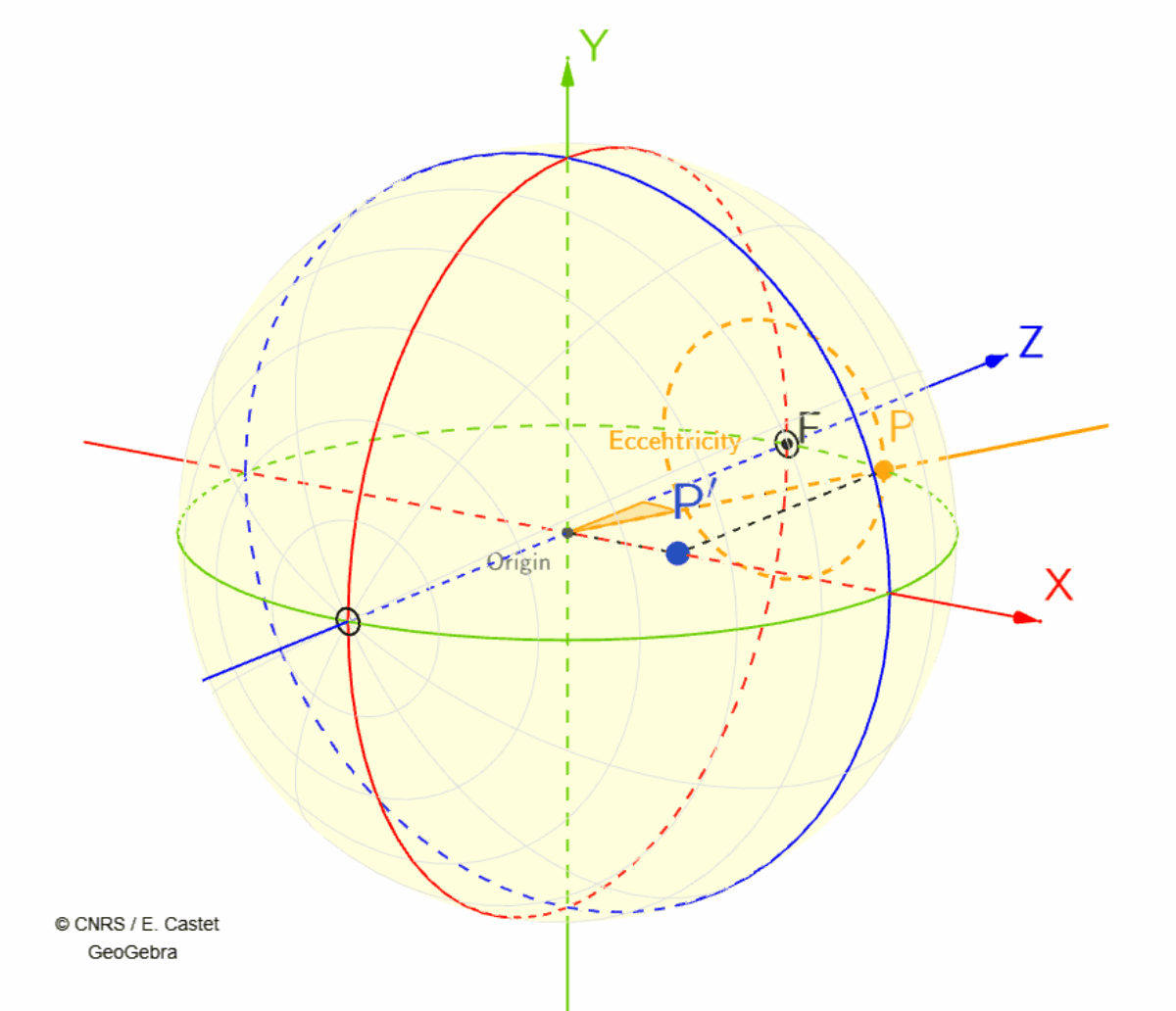Figure 4: Half-meridian of point P varies from 0° to 360° while keeping eccentricity constant at 20°.

# Flat Tangent Screen